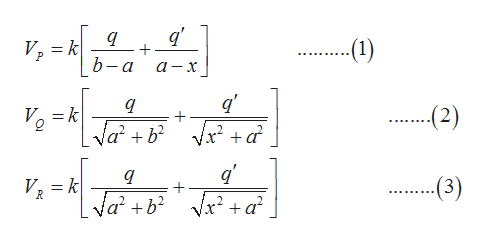Question
179 views

Consider the configuration shown in the figure: An in- finite plane conductor has a small hemispherical knob of radius a as indicated. A point charge q is placed directly to the right hand side of the hemispherical knob at a distanceb from its center. Assume that the boundary, i.e. the in- finite plane with the knob, is kept at zero potential. Find the potential V at a generic point P on the right hand side.

check_circle

Step 1

In the diagram given below, q’ is the image charge at distance x from the origin. Consider three points P, Q and R on the conductor as shown in the diagram.

Step 2

Find the potential at these three points P,Q and R and set that equal to zero(boundary condition must satisfy) and solve for distance x.help_outlineImage Transcriptionclose(1) = k} b-а VP а — х q --2) V=k Va2b2 /x? +а V, = k Va2b --(3) уx? + а? fullscreen
Step 3

Note that equation (2) and (3) are same, So, plug 0 for Vp and VQ­ in both the equat...

### Want to see the full answer?

See Solution

#### Want to see this answer and more?

Solutions are written by subject experts who are available 24/7. Questions are typically answered within 1 hour.*

See Solution
*Response times may vary by subject and question.
Tagged in

### Physics